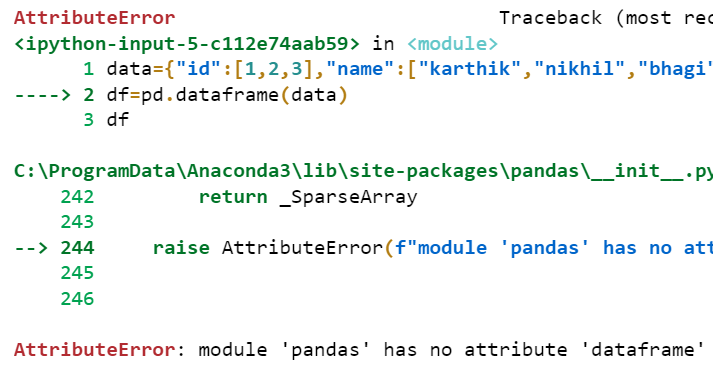# How to Fix: module ‘pandas’ has no attribute ‘dataframe’

• Last Updated : 19 Dec, 2021

In this article, we are going to see how to fix errors while creating dataframe ” module ‘pandas’ has no attribute ‘dataframe’”.

## Fix error while creating the dataframe

To create dataframe we need to use DataFrame(). If we use dataframe it will throw an error because there is no dataframe attribute in pandas. The method is DataFrame(). We need to pass any dictionary as an argument. Since the dictionary has a key, value pairs we can pass it as an argument. Dataframe considers keys as attributes and pairs as tuples. Let us see why we get errors while creating a dataframe.

## Python3

 `import` `pandas as pd ` ` `  `data ``=` `{``"id"``: [``1``, ``2``, ``3``], ` `        ``"name"``: [``"karthik"``, ``"nikhil"``, ``"bhagi"``]} ` ` `  `df ``=` `pd.dataframe(data) ` `print``(df) `

Output:To fix the above error we need to use DataFrame instead of dataframe.

## Python3

 `import` `pandas as pd ` ` `  `data ``=` `{``"id"``: [``1``, ``2``, ``3``], ` `        ``"name"``: [``"karthik"``, ``"nikhil"``, ``"bhagi"``]} ` ` `  `df ``=` `pd.DataFrame(data) ` `df `

Output:

```id        name
0    1        karthik
1    2        nikhil
2    3        bhagi```

In this way, we can fix the module ‘pandas’ has no attribute ‘dataframe’  error .

My Personal Notes arrow_drop_up
Recommended Articles
Page :